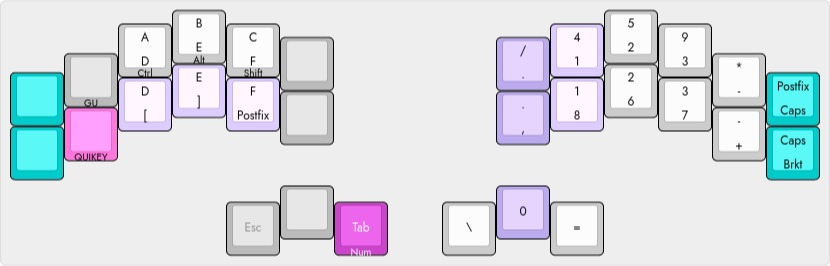# chording engine macros

tuesday, 20 april 2021

in case anyone else gets the chording engine bug, this is another example of the M() macro for DennyTom’s chording engine adding some dynamic features to the left hand hexpad of the..

## number layertoggle action
Postfix G -> “ “
Caps Upper case HEX (retoggle to disable CapsLock on exiting layer)
Brkt Square -> Round -> Curly brackets

## m() macro

the M() macro accepts 3 mandatory parameters of type const..

`M ( function, value1, value2 )`

where function is the name of the function supplied in the extra_code section of the json file and value are uint16_t const values, typically keycodes or layer references.

This structure works for the leader capitalization, passing the leader characters to the cap function which completes the action with a one shot layer to capitalize the next character.

But for dynamic keycode assignments, static variables must be buried inside the M() macro as they cannot be passed as parameters. For example, for the postfix action to toggle between a G and Space with macros M(toggle, 0, 0) and M(hexpad, 0, 0)..

`static uint16_t postfix = KC_G;` ` ` `void toggle(const struct Chord* self) {` ` switch (*self->state) {` ` case ACTIVATED:` ` postfix = postfix == KC_G ? KC_SPC : KC_G;` ` default:` ` break;` ` }` `}` ` ` `void hexpad(const struct Chord* self) {` ` switch (*self->state) {` ` case ACTIVATED:` ` key_in(KC_LSFT);` ` tap_key(postfix);` ` key_out(KC_LSFT);` ` default:` ` break;` ` }` `}`

## code space

each function declaration generates code for parameter passing. By using the value parameters of the M() macro call, a single macro can be used to toggle the dynamic hexpad states and output keycodes..

value1 value2 action command
toggle 0 1 G -> “ “ M(hexpad, 0, 1)
0 2 Square -> Round -> Curly brackets M(hexpad, 0, 2)
0 3 Upper case HEX M(hexpad, 0, 3)
output 1 0 G or “ “ M(hexpad, 1, 0)
2 1 Left bracket M(hexpad, 2, 1)
2 2 Right bracket M(hexpad, 2, 2)

saving precious EPROM space and the need to declare 6 unique macros, whilst rendering more obvious functional keycode relationships in the Number Layout..

`"type": "chord_set",` `"set": "rows",` `"keycodes": [` ` " ", " ", "A", "B", "C", " ", "/" , "4", "5", "9", "*", "M(hexpad, 0, 1)",` ` " ", "LGUI", "KM(D, LCTL)", "KM(E, LALT)", "KM(F, LSFT)", " ", "." , "1", "2", "3", "-", "M(hexpad, 0, 3)",` ` " ", " ", "M(hexpad, 2, 1)", "M(hexpad, 2, 2)", "M(hexpad, 1, 0)", " ", "," , "8", "6", "7", "+", "M(hexpad, 0, 2)",` ` "DF(BEAKL)", " ", " ", "\\", "0", "="` `]`

Using switch statements for the above..

`static uint16_t postfix = KC_G;` `static uint8_t postcap = 0;` `static uint16_t pairs[] = { ` ` {KC_NO, KC_LBRC, KC_RBRC}, ` ` {KC_LSFT, KC_9, KC_0}, ` ` {KC_LSFT, KC_LCBR, KC_RCBR}` `};` `static uint8_t bracket = 0;` ` ` `void output(int16_t modifier, int16_t keycode) {` ` key_in(modifier);` ` tap_key(keycode);` ` key_out(modifier);` `}` ` ` `void hexpad(const struct Chord* self) {` ` switch (*self->state) {` ` case ACTIVATED:` ` switch (self->value1) {` ` case 0:` ` switch (self->value2) {` ` case 1:` ` postfix = postfix == KC_G ? KC_SPC : KC_G;` ` break;` ` case 2:` ` bracket = (bracket == 0) ? 1 : ((bracket == 1) ? 2 : 0);` ` break;` ` case 3:` ` tap_key(KC_CAPS);` ` postcap = !postcap;` ` }` ` break;` ` case 1:` ` output(postcap ? KC_NO : KC_LSFT, postfix);` ` break;` ` case 2:` ` output(pairs[bracket], pairs[bracket][self->value2]);` ` }` ` }` `}`

Note: M(hexpad, 0, 3) is defined instead of simply using KC_CAPS alone, in order to produce the upper case postfix G regardless of the CapsLock state.

## extra code

combined with the leader capitalization function then, newlines represented with “\n” in the json file..

`"extra_code": "void cap(const struct Chord* self) {\n switch (*self->state) {\n case ACTIVATED:\n tap_key(self->value1);\n tap_key(self->value2);\n break;\n case DEACTIVATED:\n current_pseudolayer = CAPS;\n *self->state = IN_ONE_SHOT;\n break;\n case FINISHED:\n case PRESS_FROM_ACTIVE:\n current_pseudolayer = CAPS;\n a_key_went_through = false;\n break;\n case RESTART:\n if (a_key_went_through) {\n current_pseudolayer = self->pseudolayer;\n } else {\n *self->state = IN_ONE_SHOT;\n }\n }\n}\n\nstatic uint16_t postfix = KC_G;\nstatic uint8_t postcap = 0;\nstatic uint16_t pairs[](http://thedarnedestthing.com/3) = { {KC_NO, KC_LBRC, KC_RBRC}, {KC_LSFT, KC_9, KC_0}, {KC_LSFT, KC_LCBR, KC_RCBR} };\nstatic uint8_t bracket = 0;\n\nvoid output(int16_t modifier, int16_t keycode) {\n key_in(modifier);\n tap_key(keycode);\n key_out(modifier);\n}\n\nvoid hexpad(const struct Chord* self) {\n switch (*self->state) {\n case ACTIVATED:\n switch (self->value1) {\n case 0:\n switch (self->value2) {\n case 1:\n postfix = postfix == KC_G ? KC_SPC : KC_G;\n break;\n case 2:\n bracket = (bracket == 0) ? 1 : ((bracket == 1) ? 2 : 0);\n break;\n case 3:\n tap_key(KC_CAPS);\n postcap = !postcap;\n }\n break;\n case 1:\n output(postcap ? KC_NO : KC_LSFT, postfix);\n break;\n case 2:\n output(pairs[bracket](http://thedarnedestthing.com/0), pairs[bracket](http://thedarnedestthing.com/self->value2));\n }\n }\n}\n",`

See Json Source

»»  georgi wi